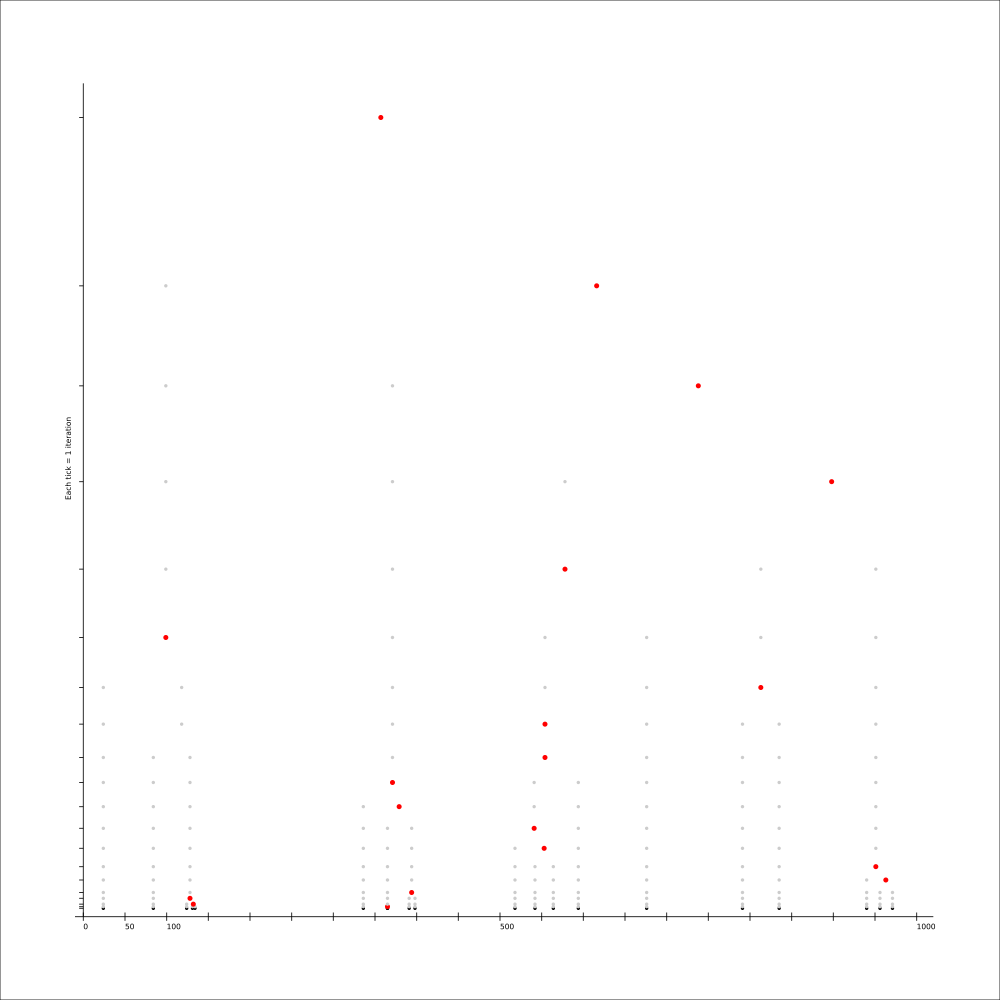Michel Albert

Fri 12 September 2014

Filed under programming

While I first intended to create the matrices manually, it turned out to take too much time if I wanted to reproduce the exact same error as happened in the unit-tests.

So in order to create the matrices used in the earlier blog-posts, I used the script at the end of this article. I took care to double-check the results however. There are times, where there's a choice of more than one cluster to pick. And as the order is not important, this choice becomes dependent on the concrete implementation, and thus non-deterministic in the unit-tests. This caused a discrepancy in the matrices generated by the script below, and the current implementation of the algorithm in library. If that was the case, I validated the results manually, and they checked out.

Unfortunately though, the data in the existing unit-test do not provoke a difference between UCLUS and average linkage (there are no real outliers). Which is unfortunate. I will (for now) not create a new data-set to verify the difference between UCLUS and average. I trust the current implementation enough to say that it will do the right thing...

Finally, here's the script used to create the test-data:

```from __future__ import print_function
from difflib import SequenceMatcher

def mean(numbers):
"""
Returns the arithmetic mean of a numeric list.
see: http://mail.python.org/pipermail/python-list/2004-December/294990.html
"""
return float(sum(numbers)) / float(len(numbers))

def median(numbers):
"""
Return the median of the list of numbers.
see: http://mail.python.org/pipermail/python-list/2004-December/294990.html
"""

# Sort the list and take the middle element.
n = len(numbers)
copy = sorted(numbers)
if n & 1:  # There is an odd number of elements
return copy[n // 2]
else:
return (copy[n // 2 - 1] + copy[n // 2]) / 2.0

def textsim(x, y):
sm = SequenceMatcher(lambda x: x in ". -", x, y)
return 1 - sm.ratio()

def simplesim(x, y):
return abs(x-y)

# sim = textsim
sim = simplesim

def single(a, b):
return min([sim(ex, ey) for ex in a for ey in b])

def complete(a, b):
return max([sim(ex, ey) for ex in a for ey in b])

def uclus(a, b):
return median([sim(ex, ey) for ex in a for ey in b])

def average(a, b):
return mean([sim(ex, ey) for ex in a for ey in b])

data = [['Lorem'],
['ipsum'],
['dolor'],
['sit'],
['amet'],
['consectetuer'],
['elit'],
['Ut'],
['elit'],
['Phasellus'],
['consequat'],
['ultricies'],
['mi'],
['Sed'],
['congue'],
['leo'],
['at'],
['neque'],
['Nullam']]

data = [[_] for _ in [791, 956, 676, 124, 564, 84, 24, 365, 594, 940, 398, 971,
131, 365, 542, 336, 518, 835, 134, 391]]

def matrix(data):
for i, row in enumerate(data):
for j, cell in enumerate(data[:i]):
yield (minsim, (i, j))

def step(data):
while len(data) > 1:
sorted_matrix = sorted(matrix(data), key=lambda x: x)
closest_items = sorted_matrix
a, b = closest_items
new_element = data.pop(max(a, b)) + data.pop(min(a, b))
data.append(new_element)
return data

def print_matrix2(data, iteration):
values = []
for i, row in enumerate(data):
simdata = [linkage(row, x) for x in data[:i]]
values.extend(simdata)
if values:
smallest = min(values)

print('.. csv-table:: Matrix #{}'.format(iteration))
print('    :stub-columns: 1')
print('    :delim: :\n')
print('    :' + ': '.join([', '.join([str(x) for x in _]) for _ in data]))
for i, row in enumerate(data):
simdata = [linkage(row, x) for x in data[:i]]
strsimdata = ['**{:.3f}**'.format(_)
if _ == smallest else '{:.3f}'.format(_)
for _ in simdata]
foo = ','.join([str(_) for _ in row])
bar = ': '.join(strsimdata)
print('    ' + foo, bar, sep=': ')

i = 1
while len(data) > 1:
print_matrix2(data, i)
print('\n')
step(data)
i += 1
print('\n')
print_matrix2(data, i)
print('\n')
```

Wed 10 September 2014

Filed under programming

Here we go again...

After further analysis (the trial & error kind), I found out that the error of modified values only occurs in unsorted data. Which makes perfect sense as Python's list.remove picks the first matching item. So the ordering of data plays a role.

As a quick fix …

Tue 09 September 2014

Filed under programmingThis is a thumbnail of a visualisation of the separate clustering steps. Open original SVG file for better readability.

The linked image shows the individual steps through the existing clustering algorithm. Each "horizontal line" (the Y-Axis) represents one step of the algorithm. The distance between each "line" represents the level …